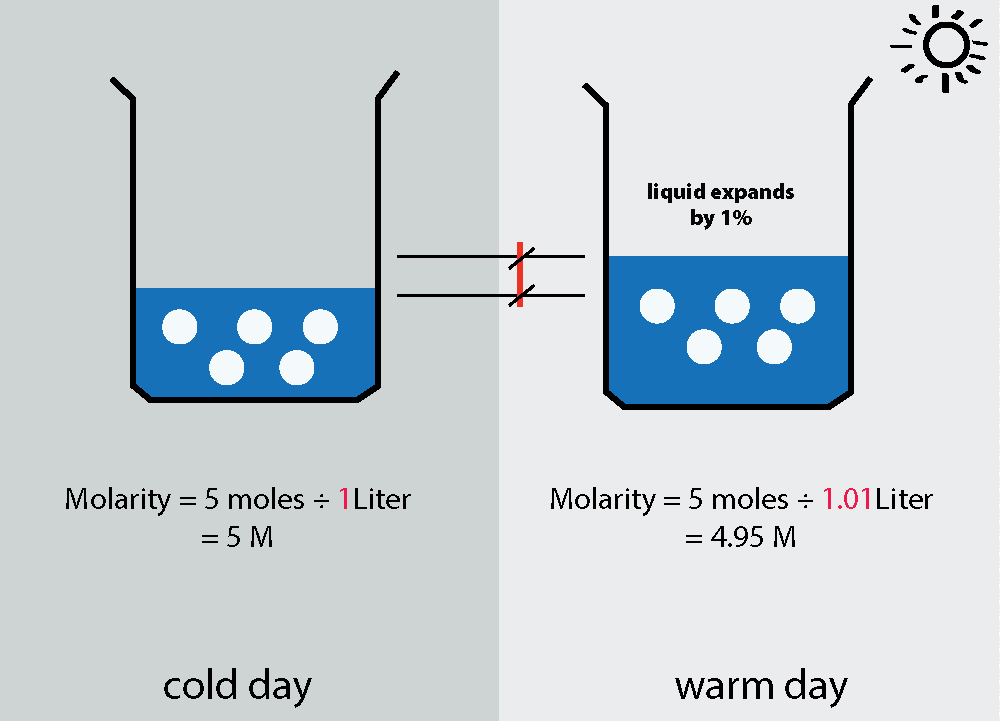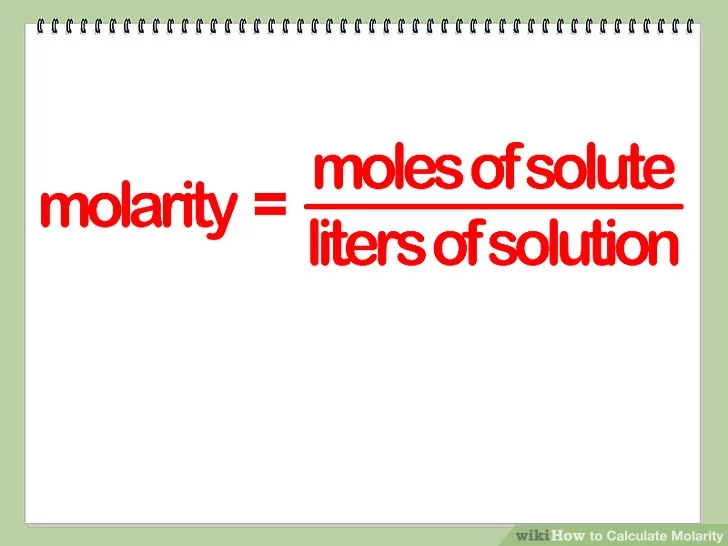# Molarity Study Guide

INTRODUCTION

Every system needs a measuring unit; for example, when your teacher asks you to draw a line of 5 units, you will ask her the scale to draw a line of 5 m or 5 cm or any other unit. Similarly, you will need to know the concentration of substances mixed with a solvent to get the desired solution. Hence, the invention of molarity took place.Source

### WHAT IS MOLARITY?

Molarity definition in chemistry refers to the number of moles present in a volume of solution in liters. Different elements react together in ratios of the whole number; their volume is expressed in moles. Molarity is the concentration unit and helps find out the concentration of different substances present in the solution.

### MOLARITY FORMULA

It is straightforward to find out the molarity; you need to know the solute’s moles and the solution’s volume. The formula of molarity is:

``                                    M = n / V``

Here,

M = the molality of the solution that is to be calculated

n = the number of moles of the solute

V = the volume of solution given in terms of litersSource

### EXAMPLE PROBLEMS

A solution is prepared by bubbling 2.56 grams of hydrochloric acid in water. Here, the volume of the solution is 46.8 mL. Calculate the molarity of the solution.

Solution:

The chemical formula of hydrochloric acid = HCl

The chemical formula for water = H₂O

The molecular weight of HCl = 35.5 × 1, 1 × 1 = 36.5 moles/gram

The molecular weight of H₂O = 1 × 2, 16 × 1 = 18 moles/gram

Given, the mass of hydrochloric acid in the solution = 2.56 g

The number of moles of hydrochloric acid = mass in gm / molecular weight = 2.56 / 36.5 = 7.02 x 10⁻² mole

Now, given the volume of the solution = 46.8 mL

Expressing the volume in terms of liters,

Volume = 46.8 / 1000 = 4.68 x 10⁻² L

∴ molarity of the substance = number of moles of elements/volume of the solution in liters = 7.02 x 10-2 / 4.68 x 10⁻² = 1.5 M

Ans: The molarity of the solution is 1.5 M

### FAQs:

1. What is a molar solution? Give an example.

Ans: The molar solution is the gram molecular weight of the solute used to calculate the molar concentration in liters of the solution. Hence, 1 molar solution (M) will contain 1.0 GMW of a substance dissolved in the solvent to make 1 liter of the solution. For example:The 1 M solution of NaCl will contain 58.44 g.

2. How do I calculate molarity?

Ans: To calculate the molarity of a solution, follow these simple steps:

Step 1: Find out the number of moles of solute present in the solution.

Step 2: Then, find out the volume of the solution in liters.

Step 3: Finally, divide the moles of solute with liters of solution, and you willget molarity.

3. What is the molarity of a solution?

Ans: The molarity of a solution can be defined as the moles of a solute per liter of the solution. In simple words, it is the amount of a substance present in a certain volume of a solution.

4. How is molarity used in everyday life?

Ans: The molarity is used to study the different properties of solutions when subjected to vapor pressure or temperature changes.

We hope you enjoyed studying this lesson and learned something cool about Molarity: Definition and Example! Join our Discord community to get any questions you may have answered and engage with other students like you! Don’t forget to download our app to experience our fun VR classrooms – we promise it makes studying much more fun! 😎

## SOURCES:

1. Calculate Molarity of Solution: https://www.thoughtco.com/calculate-molarity-of-a-solution-606823. Accessed 28 Feb 2022.
2. What is Molarity? https://sciencetrends.com/what-is-molarity-with-examples/#:~:text=Molarity%20is%20how%20chemists%20measure,a%20given%20amount%20of%20solution. Accessed 28 Feb 2022.
]]>